#### Critical Random Graphs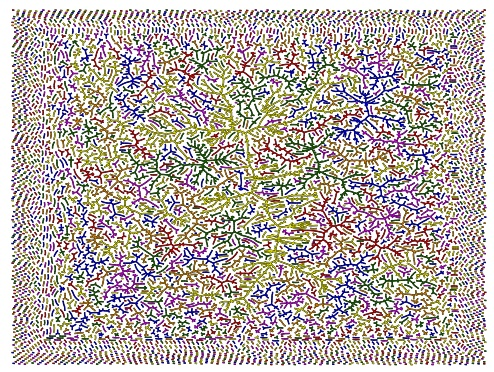Recently, I've spent some time thinking about the structure of critical Erdos--Rényi random graphs $G(n,p)$ inside the critical window, i.e., with $p=\frac 1 n + \lambda n^{-4/3}$ for some $\lambda\in \mathbb R$.

With Louigi Addario and Christina Goldschmidt, we proved that one can define a non-trivial scaling limit for the entire sequence of connected components when the distances are multiplied by $n^{-1/3}$.

Aldous had already proved that the sequence of sizes and surpluses of the connected does have a limit that can be described in terms of a Brownian motion with parabolic driff. The limit object for the graph itself is a sequence of random metric spaces that is actually encoded in the original Brownian with parabolic drift used by Aldous. The connected components may be constructed via tilting the distribution of Aldous' continuum random tree, and adding a number of point identifications to create cycles. The picture which looks like the antique persian rug tries to illustrates this convergence. It is $G(n,p)$ with $n=40000$ and $p=1/n$. No wonder the large components are tree-like! You will probably see a little more clearly on the pdf picture.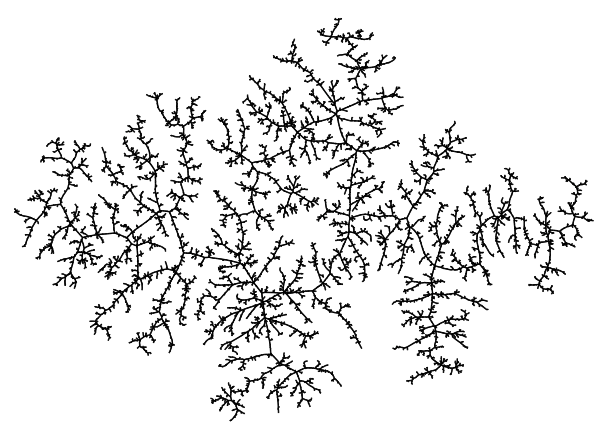I've also extracted the largest component. Each connected component is a biased CRT where some of the vertices have been idenfied. The contour function of a canonical spanning tree is not exactly a Brownian excursion, it is biased by the area.

To get a decent chance to have some cycles in the largest component, I picked $p=\frac 1n+n^{-4/3}$. The sample picture here has $n=40000$. See also the pdf picture.

Details of the constructions are available in two papers that can be found here, or at [arXiv:0903.4730] and [arXiv:0908.3629].

#### Random Mean-Field Minimum Spanning Tree

The minimum spanning tree is one of the important objects in combinatorial optimization. Take a complete graph on n vertices, with edges weighted by i.i.d. random variables uniform on $[0,1]$. Build the minimum spanning tree with your favorite algorithm, and observe what it looks like. Here is an example, with $100000$ nodes. The PDF picture can be downloaded from here (although it's probably unnecessarily huge...).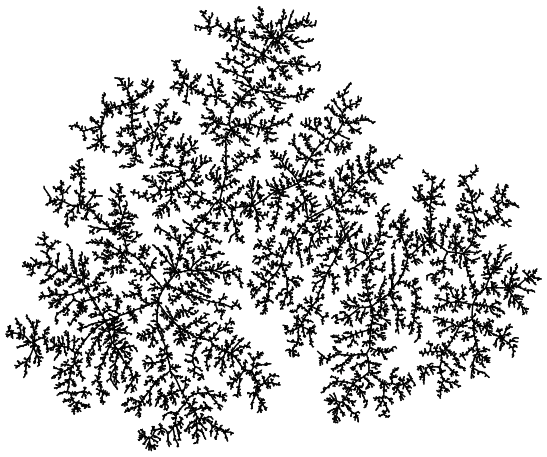#### Random Euclidean MST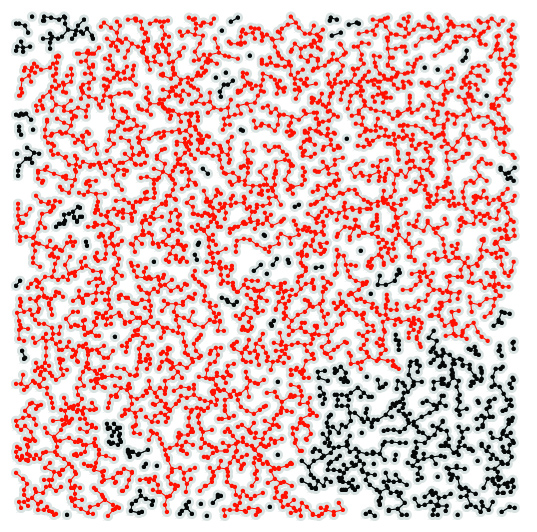Here is couple of really pretty simulation images. I was wondering about some parameters the merging process when running Kruskal's algorithm on a random geometric graph: take a n random uniform points in the unit square, connect two points if the distance between them is less than r.

For a suitable scaling of $r$ and $n$, you then obtain a picture similar to the one shown on the right: a random Euclidean graph over $2000$ uniform points in the unit square. The corresponding and the corresponding minimum spanning forest is shown on Figure 2, together with the largest component in red.

These were done when trying to obtain information about the tree explaining Kruskal: the binary tree explaining the way the components get merged by the algorithm. The parameter I was looking for was the height of this tree. It turns out that the result is not too difficult to prove, but the answer is quite surprising... Take a guess if you want. I should post the answer as soon as the paper we are writing with Luc and Erin is submitted. These are JPG pictures exported from the initial scale-free PS files. You can get the vector based PDF files here.

#### Random Geometric Graphs and Irrigations Graphs

Random geometric graphs are great models for mobile networks and sensor networks. For applications, one would like networks to be connected (of course), and as sparse as possible.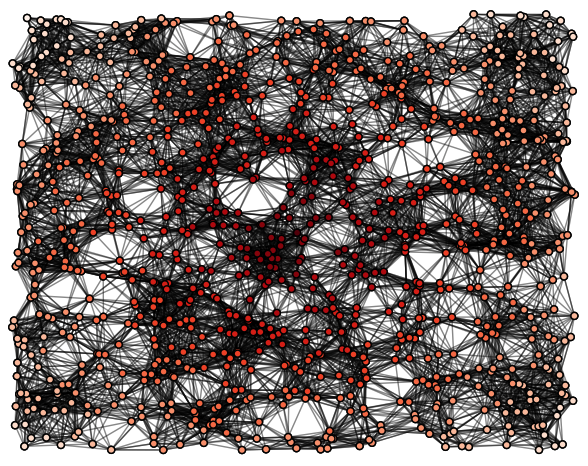Random geometric graphs $G(n,r)$ are typically connected when the expected number of points in balls of radius $r$ is of order $\log n$, i.e, when the number of neighbours of typical nodes is of order $\log n$. Although this is not enormous, it might still be too large for some applications involving very light devices. Here, a random geometric graph $G(400,0.2)$ on $400$ uniform points in the unit square.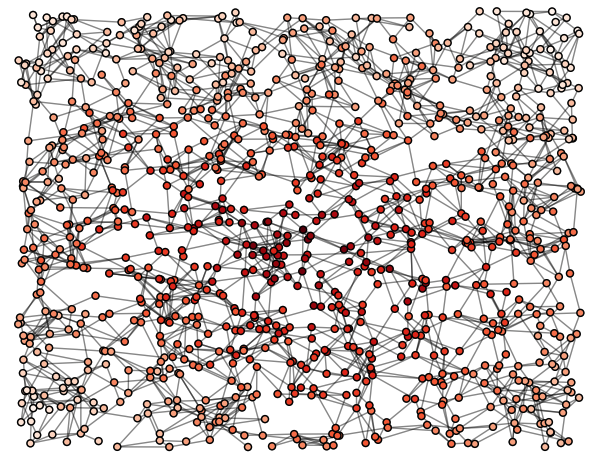One way to cope with this issue is to further sparsify the graph. One natural way to do this is to fix a number $c(n)$, and keep only $c(n)$ random (out-)neighbours. The sparsified graph is then called an irrigation graph. It turns out that for $nr^{2}=\gamma \log n$, i.e. around the threshold for connectivity, taking $$c(n)\ge\sqrt{\frac{2 \log n}{\log \log n}}$$ is (essentially) necessary and sufficient for the sparsified graph to be connected. This is proved in the paper Connectivity thresholds for Bluetooth graphs'' that we wrote with L. Devroye, G. Lugosi and N. Fraiman available on my research page. The following picture shows the sparsification of the random graph $G(400,0.2)$ on the previous picture, keeping only $c=2$ edges out for each node.

One can actually show a much nicer result: if one only keeps on average $(1+\epsilon)$ neighbour per node, then the sparsified graph contains a giant component of $n-o(n)$ nodes. This is almost optimal, since any such giant requires $n-o(n)$ edges, and still it does not involve any global calculation and is fully distributed !